Octet expansion and hypervalence in dimethylidyne-λ6-sulfane.

I started this story by looking at octet expansion and hypervalence in non-polar hypercoordinate species such as S(-CH3)6, then moved on to S(=CH2)3. Finally now its the turn of S(≡CH)2.As the triple bonds imply, this seems to represent twelve shared valence electrons surround the sulfur, six from S itself and three from each carbon. The octet is clearly expanded from eight to twelve. But is all as it seems?

The linear form reveals the following localized orbitals. Six NBOs are localized to the S-C regions, of which four are bonding, two σ and two π. The remaining four electrons are in two non-bonding lone pairs, with a mild anti-bonding S-C component. So the bond order comes out as ~four, not six! This corresponds to the story told in the earlier blogs that the electrons in excess of the octet tend to occupy either non or antibonding orbitals.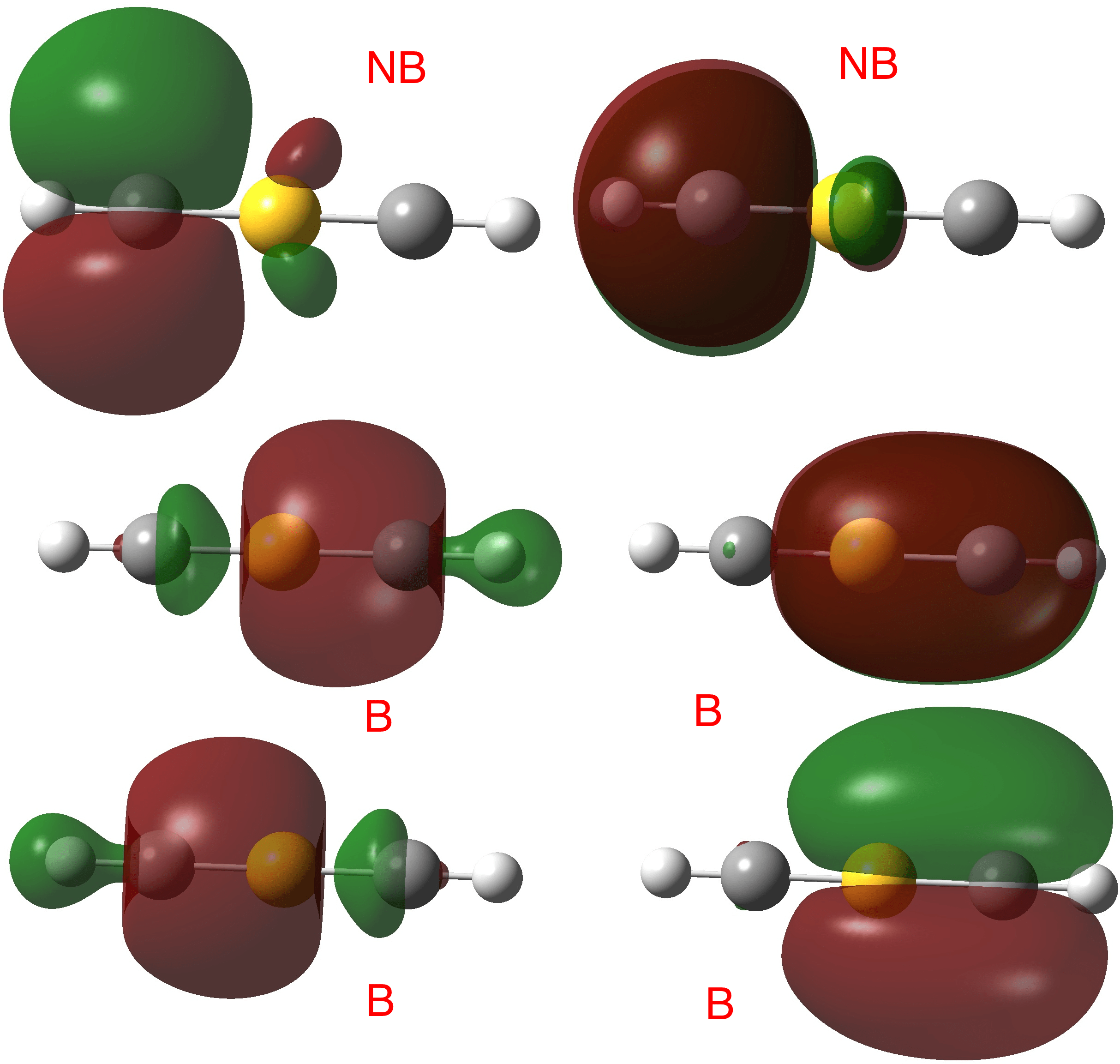In fact the full NBO analysis gives a value of 4.0920 for the S bond index and little Rydberg character; S: [core]3S(1.02)3p(3.61)3d(0.13).

Next, the ELF analysis, based not on orbitals but the derived electron densities. Each S-C region shows an ELF circular attractor integrating to 5.44e (or 10.88e for the S valence region). So the ELF reflects not only the density arising from bonding orbitals, but the non-bonding ones as well!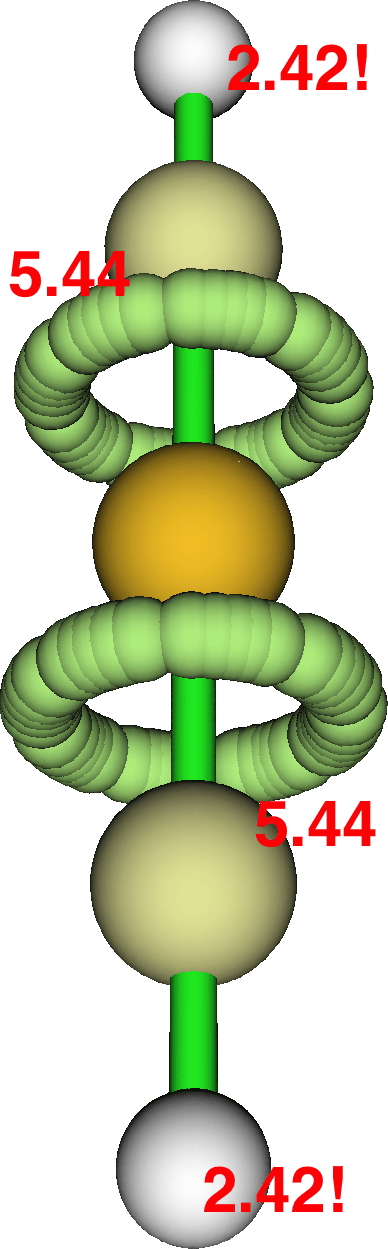Take a look at the ELF basin for the two hydrogen atoms; at 2.42e each this shell is ALSO expanded from the normal 2! Apart from the normal C-H localised NBO orbital, one can also see small C-H bonding contributions from the four NBOs labelled B above as well. So ELF analysis of the shared electrons in this species seems to show octet expansion for S and similar shell expansion for H. But we now know that simply taking the ELF basin population and dividing by two to get the bond or valence index can be misleading. The ELF analysis includes non or even anti-bonding density contributions and so it cannot be used to infer hyperbonding (hypervalence).

I must now confess to withholding some vital information from you. The linear HC≡S≡CH molecule is not a minimum, having four computed negative force constants, the normal mode of one of which is animated  below.The true minimum has C2 symmetry as follows and it corresponds to that mysterious structure shown at the top and hitherto not mentioned. This form is 14.6 kcal/mol lower in free energy than the linear variety.The ELF analysis confirms this species as bis(carbene), with two “lone pairs” on S. All the octet expansion has vanished; of the ~six electrons hitherto located in each C-S region, four have morphed into lone pairs, leaving only ~two in the S-C regions. The sulfur is now allocated 7.44e, a  “normal” octet.At this point, I remind that the great G. N. Lewis himself, the original coiner of the eight electron valence rule, pondered whether acetylene might have a related bis(carbene) form. It is nice to come up with an example of this more than 100 years after his original suggestion.

FAIR Data DOI for the collection: 10.14469/hpc/3333

3 Responses to “Octet expansion and hypervalence in dimethylidyne-λ6-sulfane.”

1.Henry Rzepa says:

Here is an “experiment” in removing the four non-bonding electrons from linear HC≡S≡CH to reduce the valence total being considered from 12 to 8. This is achieved by replacing S by Be. The resulting DFT-based molecular orbitals (ωB97XD/Def2-TZVPP) are shown below for this linear form. The two at the bottom represent the H-C bonds, and the four top ones are the eight valence electrons associated with Be. These comprise two σ-type S-C orbitals and two π-type degenerate MOs.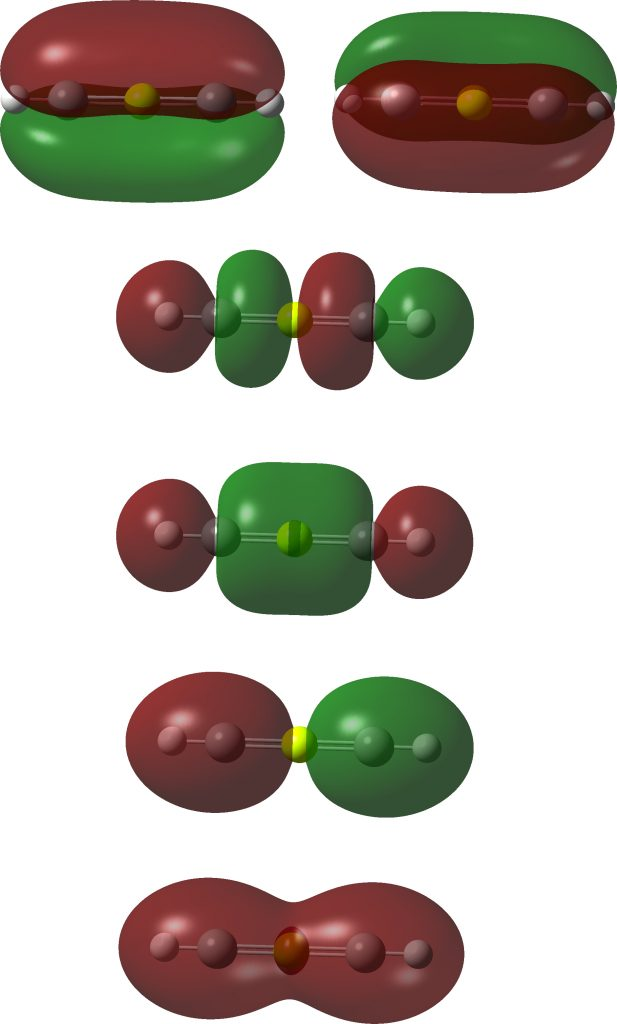Next, the localized versions. The bottom two are localized C-H NBOs, the top two are three-centre π-levels. The middle two are supposed to be the Be-C σ-NBOs, but some odd asymmetry has crept in. This may be a sign of an “unstable” wavefunction, often caused by a significant level of multi-reference character. The NBO analysis is equally strange. The Be bond index is 0.96, whilst the two carbons are 3.34 each. But the next bit is really unusual. The C-Be Wiberg bond order is only 0.48 (0.96/2) whereas the C-C bond order is 1.88! This for two atoms that are 3.04Å apart.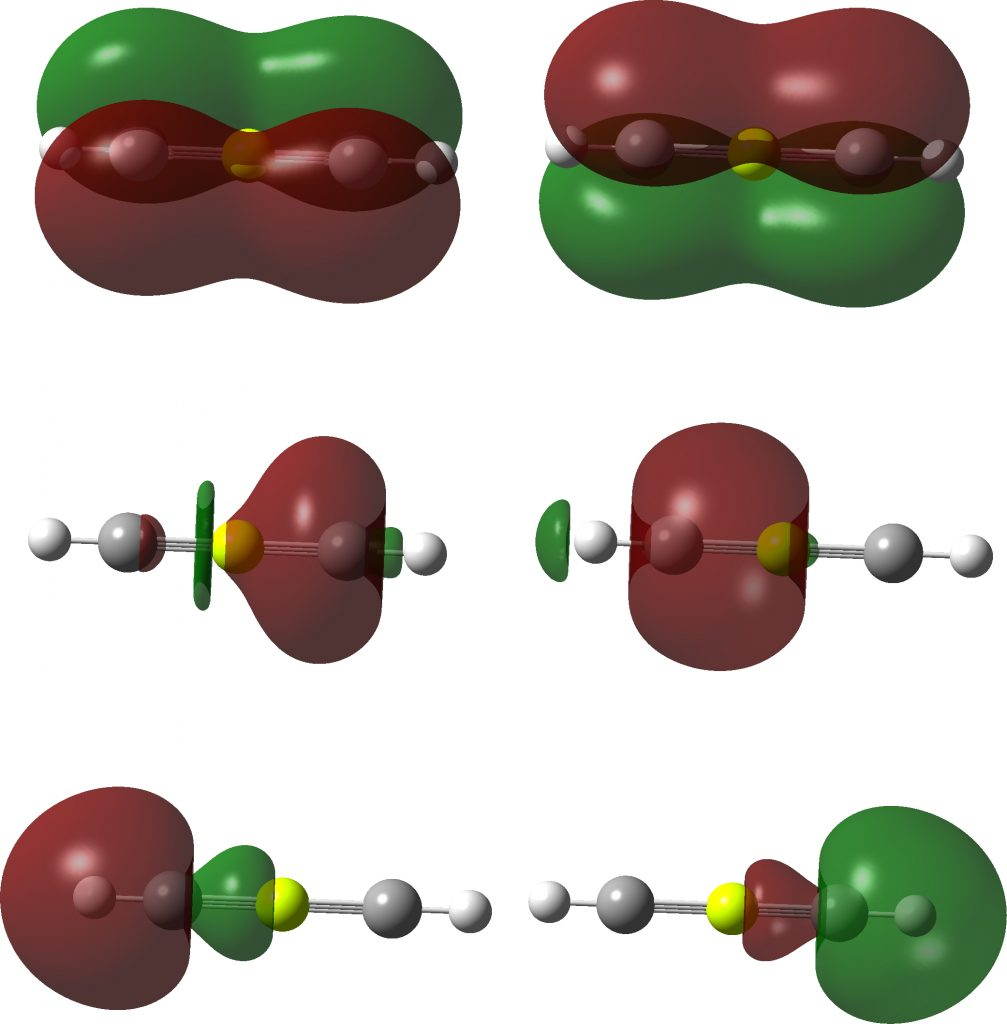Next the ELF analysis at the DFT level. This reveals circular ELF attractors in the C-Be regions of 3.62e each, and 2.27e for the C-H basin. This is very much at odds with the apparent C-Be bond orders, and again we see that simply taking an ELF basin population and trying to map that to valence electrons can fail badly.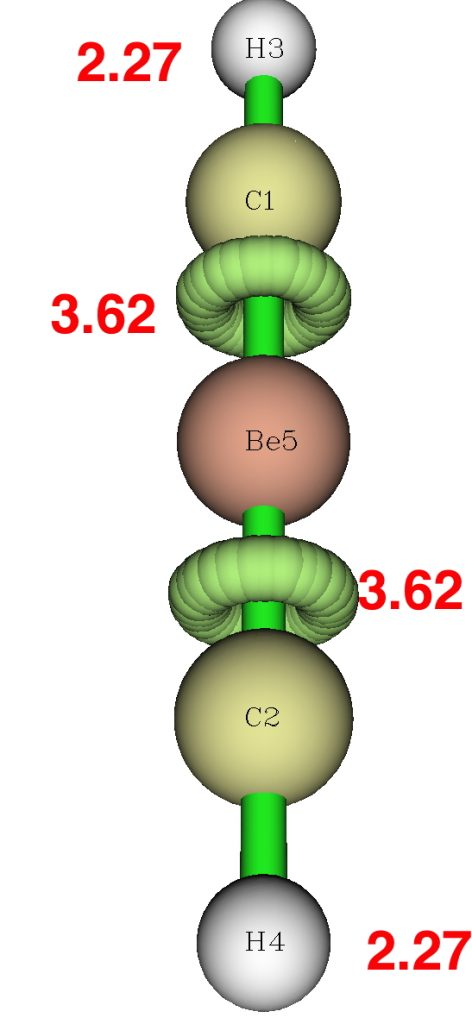Because the wavefunction analysis is so odd, I repeated using an aforementioned multireference wavefunction, using CASSCF(12,12) (this stands for an active orbital space of 12, populated by 12 electrons). NBO analysis of such a wavefunction is generally not recommended, so only the ELF is shown.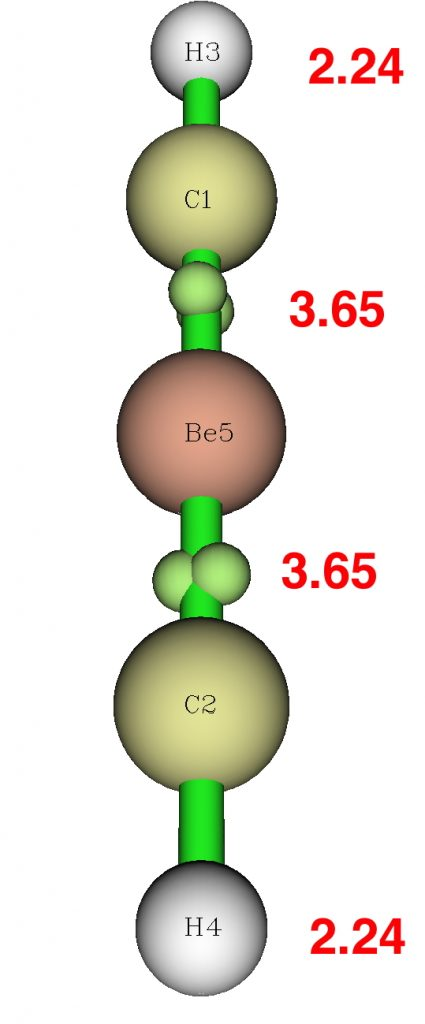The CASSCF(12,12) wavefunction shows significant contributions from excited configurations, which maps to significant non-integer natural orbital occupancies (1.3684663*2, 0.6331417*2).

Oh, as before, the linear form is not a true geometrical minimum in energy, but distorts in the same manner as did the S form.

2.Mike Turner says:

Is NSN a minimum? Is it linear? Where are the electrons?

3.Henry Rzepa says:

The analysis for N≡S≡N is shown below. The excess valence electrons surrounding S (12 at the start) have fled into the nitrogen non/anti-bonding regions, and so sulfur here is not octet-exceeded.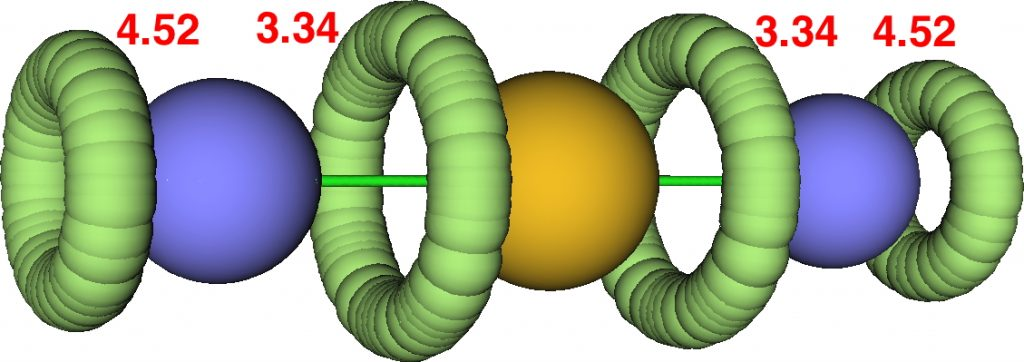The FAIR data is at 10.14469/hpc/3387.